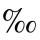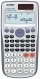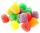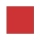Division

Divide by the number 0.2 is the same as multiply by what number?

Result

x =  5

Solution:Leave us a comment of example and its solution (i.e. if it is still somewhat unclear...):Be the first to comment!Next similar examples:

1. Double 5Peter was thinking of a number. Peter doubles it and gets an answer of 8.6. What was the original number?
2. EvaluateThe division of numbers 18 and 6 increase by product of the numbers 156 and 0.001
3. On the tripOn the trip were more than 55 children, but less than 65 children. Groups of 7 could be formed, but groups of 8 no. How many children were on a trip?
4. Quotient 3If the quotient of 8/13 and 2 is subtracted from the product of 1 3/4 and 8/21, what is the difference?
5. SymbolsIf 2*3 = 60 ; 3*4 = 120 and 4*5 = 200, what is 2*5?
6. PromileCalculate the 4.6 ‰ of 199.
7. CustomerA customer purchase three (3) writing pads from a store. She receive k9.70 change from a k10.00 note . How much was it's writing pad cost?
8. SickSick Marcel already taken six tablets, which was a quarter of the total number of pills in the pack. How many pills were in the pack?
9. To improper fractionChange mixed number to improper fraction a) 1 2/15 b) -2 15/17
10. Without 2Without multipying, tell whether the product 0.644 x 0.25 will be greater than 1 or less than 1? Explane how you know. Then find the product.
11. FractionDetermine for what x fraction ?:
12. Equation 11Solve equation: ?
13. ChocolateI eat 24 chocolate in 10 days. How many chocolate I eat in 15 days at the same pace?
14. MidpointsTriangle whose sides are midpoints of sides of triangle ABC has a perimeter 45. How long is perimeter of triangle ABC?
15. SimplifySimplify powers multiplication: (3+22)(5-42)
16. BicycleThe bicycle pedal gear has 36 teeth, the rear gear wheel has 10 teeth. How many times turns rear wheel, when pedal wheel turns 120x?
17. Reciprocal equation 3Solve reciprocal equation: 1/2 + 2/3=1/x